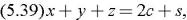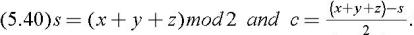Computer Arithmetic Algorithms, Second Edition

# 5.11: Carry-save adders

## 5.11 Carry-save adders

When three or more operands are to be added simultaneously (e.g., in multiplication) using two-operand adders, the time-consuming carry-propagation must be repeated several times. If the number of operands is k, then carries have to propagate ( k ?1) times. Several techniques for multiple operand addition that attempt to lower the carry-propagation penalty have been proposed and implemented. The technique that is most commonly used is carry-save addition. In carry-save addition, we let the carry propagate only in the last step, while in all the other steps we generate a partial sum and a sequence of carries separately. Thus, the basic carry-save adder (CSA) accepts three n-bit operands and generates two n-bit results, an n-bit partial sum, and an n-bit carry. A second CSA accepts these two bit-sequences and another input operand, and generates a new partial sum and carry. A CSA is therefore, capable of reducing the number of operands to be added from 3 to 2, without any carry propagation.

A carry-save adder may be implemented in several different ways. In the simplest implementation, the basic element of the carry-save adder is a full adder with three inputs, x, y, and z, whose arithmetic operation can be described bywhere s and c are the sum and carry outputs, respectively. Their values areThe outputs are the weighted binary representation of the number of 1 s in the inputs. We therefore call the...

UNLIMITED FREE ACCESS TO THE WORLD'S BEST IDEAS